Study S2 Mathematics Maths Factorising Quadratic Expressions - GeniebookIn this chapter, we will be discussing the below-mentioned topics in detail:

• Factorisation as the reverse process of Expansion
• Factorisation using a Multiplication Frame
• Factorisation using a Factorisation Table
• Factorisation involving extracting common factor and quadratic expressions

## Factorisation Of Algebraic Expressions

 Types Of Factorisation \begin{align} \xrightarrow [] {\quad \text{ Expansion }\quad } \end{align} Extract Common Factor \begin{align*} 2x(x-1) &= \end{align*} \begin{align*} 2x^2-2x \end{align*} Quadratic Factorisation \begin{align} (x-2)(x-1) &= \end{align} \begin{align} x^2-3x+2 \end{align} $$\xleftarrow{\quad \text{ Factorisation }\quad}$$

Factorisation is the opposite of expansion.

Let’s understand this with the help of some examples:

Question 1:

Factorise $$3x^2-27x$$ completely.

Solution:

The common factor is $$3x$$.

$$3x^2-27=3x(x-9)$$

Question 2:

Factorise $$x^2+5x+6$$ completely.

Solution:

Method (a): Factorisation using Multiplication Frame

$$x^2+5x+6$$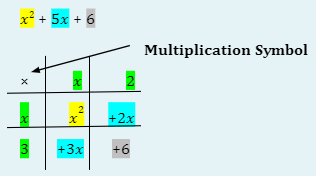As we know, $$x$$ times $$x$$ will give us $$x^2$$, and plot both the $$x$$ in Row $$I$$ Column $$II$$ and Row $$II$$ Column $$I$$, respectively.

Also, either 3 times 2 is 6 or 1 times 6 is 6, so using trial and error, put 3 in Row $$III$$ Column $$I$$ and put 2 in Row $$I$$ Column $$III$$.

Multiply Row $$I$$ Column $$III$$, i.e. 2, by Row $$II$$ Column $$I$$, i.e. $$x$$ and put $$2x$$ in Row $$II$$ Column $$III$$.

Multiply Row $$III$$ Column $$I$$, i.e. 3, by Row $$I$$ Column $$II$$, i.e. $$x$$ and plot $$3x$$ in Row $$III$$ Column $$II$$.

Now, $$+2x$$ and $$+3x$$ give us $$+5x$$

However, if we use 1 times 6 instead, see below.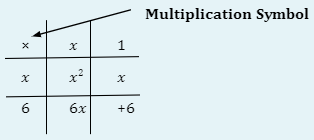Now, $$+6x$$ and $$+x$$ do not give us $$+5x$$, and it gives us $$+7x$$.

Hence, the answer would be $$(x+2)(x+3)$$

Method (b): Factorisation using Factorisation Table

$$x^2+5x+6$$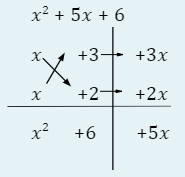$$x^2+5x+6=(x+3)(x+2)$$

Question 3:

Factorise $$x^2+6x+5$$ completely.

Solution:

Using a factorisation table,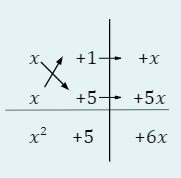$$\therefore x^2+6x+5=(x+1)(x+5)$$

Question 4:

Factorise $$3x^2-18x+15$$ completely.

Solution:

Firstly, we extract out a common factor 3.

$$3x^2-18x+15=3(x^2-6x+5)$$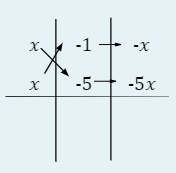\begin{align} x^2 +5 -6x \end{align}

\begin{align*} \therefore \quad 3x^2-18x+15&=3(x^2-6x+5)\\ &=3(x-1)(x-5) \end{align*}

Continue Learning
Algebraic Fractions Direct & Inverse Proportion
Congruence And Similarity Factorising Quadratic Expressions
Further Expansion And Factorisation Quadratic Equations And Graphs
Simultaneous EquationPrimaryPrimary 1Primary 2Primary 3Primary 4Primary 5Primary 6SecondarySecondary 1Secondary 2EnglishMaths
Algebraic Fractions
Direct & Inverse Proportion
Congruence And Similarity
Further Expansion And Factorisation
Simultaneous EquationScienceSecondary 3Secondary 4